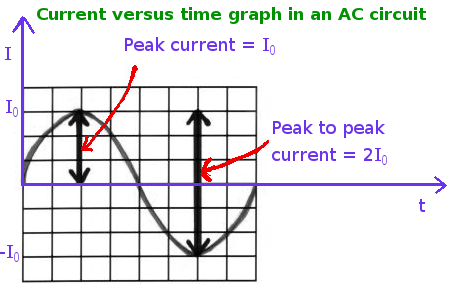# The peak to peak current passing through a 200 \Omega resistor is 22.0 A. Find the maximum...

## Question:

The peak to peak current passing through a {eq}200 \Omega {/eq} resistor is {eq}22.0 A {/eq}. Find the maximum voltage across the resistor and the RMS current through the resistor.

## AC circuit with a Resistor:

If the alternating voltage of the AC source is given by the equation;

{eq}V = V_0 \sin (\omega t ) {/eq}

then, the alternating current in the AC circuit containing only a resistor is given by the equation;

{eq}I = I_0 \sin (\omega t ) {/eq}

where,

• {eq}V_0 {/eq} is the maximum voltage,
• {eq}I_0 = \dfrac{V_0}{R} {/eq} is the maximum current and
• {eq}\omega {/eq} is the angular frequency of the voltage source.

The RMS voltage is given by

{eq}V_{RMS} = \dfrac{V_0}{\sqrt{2}} {/eq}

and he RMS current is given by

{eq}I_{RMS} = \dfrac{I_0}{\sqrt{2}} {/eq}

Become a Study.com member to unlock this answer! Create your account

A diagram showing the peak current and peak to peak current is shown in the figure 1 below.Given:

• The resistance of the resistor is...Impedance in Alternating Current Circuits

from

Chapter 13 / Lesson 8
4.1K

In this lesson, you will learn about topics that include the difference between DC and AC circuits, the use of rms values, definitions of impedance and reactance, and calculations involving the reactance of capacitors and inductors.